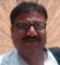## How to set python environment variable PYTHONPATH on Mac?Updated on 30-Sep-2019 08:46:51

## What is PYTHONPATH environment variable in Python?Updated on 30-Jul-2019 22:30:20
PYTHONPATH is an environment variable which you can set to add additional directories where python will look for modules and packages. For most installations, you should not set these variables since they are not needed for Python to run. Python knows where to find its standard library.The only reason to set PYTHONPATH is to maintain directories of custom Python libraries that you do not want to install in the global default location (i.e., the site-packages directory).Read the following docs to get a better understanding of Python environment variables: http://docs.python.org/using/cmdline.html#environment-variables

## How to locate a particular module in Python?Updated on 30-Sep-2019 08:48:23
For a pure python module you can find the location of the source files by looking at the module.__file__. For example,>>> import mymodule >>> mymodule.__file__ C:/Users/Ayush/mymodule.pyMany built in modules, however, are written in C, and therefore module.__file__ points to a .so file (there is no module.__file__ on Windows), and therefore, you can't see the source.Running "python -v" from the command line tells you what is being imported and from where. This is useful if you want to know the location of built in modules.

## What is the use of "from...import *" Statement in Python?Updated on 30-Sep-2019 08:48:52
The "from module import *" statement is used to import all function from a Python module. For example, if you want to import all functions from math module and do not want to prefix "math." while calling them, you can do it as follows:>>> from math import * >>> sin(0) 0.0 >>> cos(0) 1.0Note that for any reasonable large set of code, if you import * you will likely be cementing it into the module, unable to be removed. This is because it is difficult to determine what items used in the code are coming from 'module', making it easy ... Read More

## What is the use of "from...import" Statement in Python?Updated on 30-Sep-2019 08:49:27
The "from module import function" statement is used to import a specific function from a Python module. For example, if you want to import the sin function from the math library without importing any other function, you can do it as follows:>>> from math import sin >>> sin(0) 0.0Note that you don't have to prefix sin with "math." as only sin has been imported and not math. Also you can alias imported functions. For example,>>> from math import cos as cosine >>> cosine(0) 1.0

## How to import a single function from a Python module?Updated on 30-Sep-2019 08:30:55
You can use the "from module import function" statement to import a specific function from a Python module. For example, if you want to import the sin function from the math library without importing any other function, you can do it as follows:>>> from math import sin >>> sin(0) 0.0Note that you don't have to prefix sin with "math." as only sin has been imported and not math. Also you can alias imported functions. For example,>>> from math import cos as cosine >>> cosine(0) 1.0

## How to do multiple imports in Python?Updated on 30-Sep-2019 08:31:50
To import multiple modules, just use the import statement multiple times. For example, >>> import os >>> import math >>> import sysSometimes grouping imports make more sense. To import multiple modules with a single import statement, just separate the module names by commas. For example, >>> import math, sys, osIf you want to change the name under which the modules are imported, just add as after each module name followed by module alias. For example, >>> import math as Mathematics, sys as systemIf you have a list of modules you want to import as strings, then you can use the ... Read More

## How to use multiple modules with Python import Statement?Updated on 30-Sep-2019 08:32:35
To import multiple modules with a single import statement, just separate the module names by commas. For example,>>> import math, sys, osIf you want to change the name under which the modules are imported, just add as after each module name followed by module alias. For example,>>> import math as Mathematics, sys as systemIf you have a list of modules you want to import as strings, then you can use the inbuilt __import__(module_name). For example,>>> modnames = ["os", "sys", "math"] >>> for lib in modnames:  ...     globals()[lib] = __import__(lib)

## What is the use of import statement in Python?Updated on 30-Sep-2019 08:33:07
To use any package in your code, you must first make it accessible. You have to import it. You can't use anything in Python before it is defined. Some things are built in, for example the basic types (like int, float, etc) can be used whenever you want. But most things you will want to do will need a little more than that. For example, if you want to calculate cosine of 1 radian, if you run math.cos(0), you'll get a NameError as math is not defined. You need to tell python to first import that module in your code ... Read More